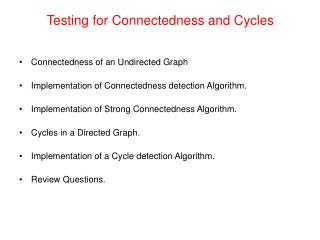Download PresentationTesting for Connectedness and Cycles# Testing for Connectedness and Cycles - PowerPoint PPT Presentation

Download Presentation##### Testing for Connectedness and Cycles

Download Policy: Content on the Website is provided to you AS IS for your information and personal use and may not be sold / licensed / shared on other websites without getting consent from its author. While downloading, if for some reason you are not able to download a presentation, the publisher may have deleted the file from their server.

- - - - - - - - - - - - - - - - - - - - - - - - - - - E N D - - - - - - - - - - - - - - - - - - - - - - - - - - -
##### Presentation Transcript

1. Testing for Connectedness and Cycles • Connectedness of an Undirected Graph • Implementation of Connectedness detection Algorithm. • Implementation of Strong Connectedness Algorithm. • Cycles in a Directed Graph. • Implementation of a Cycle detection Algorithm. • Review Questions.

2. V = {A, B, C, D, E, F} E = {{A, B}, {A, C}, {B, C}, {D, E}, {E, F}} Connectedness of an Undirected Graph • An undirected graph G = (V, E) is connected if there is a path between every pair of vertices. • Although the figure below appears to be two graphs, it is actually a single graph. • Clearly, G is not connected. e.g. no path between A and D. • G consists of two unconnected parts, each of which is a connected sub-graph --- connected components.

3. Implementation of Connectedness Algorithm • A simple way to test for connectedness in an undirected graph is to use either depth-first or breadth-first traversal - Only if all the vertices are visited is the graph connected. The algorithm uses the following visitor: public class CountingVisitor extends AbstractVisitor { protected int count; public int getCount(){ return count;} public void visit(Object obj) {count++;} } • Using the CountingVisitor, the isConnected method is implemented as follows: public boolean isConnected() { CountingVisitor visitor = new CountingVisitor(); Iterator i = getVertices(); Vertex start = (Vertex) i.next(); breadthFirstTraversal(visitor, start); return visitor.getCount() == numberOfVertices; }

4. V = {A, B, C, D, E, F} E = {(A, B), (B, C), (C, A), (B, E), (D, E), (E, F), (F, D) Connectedness of a Directed Graph • A directed graph G = (V, E) is strongly connected if there is a directed path between every pair of vertices. • Is the directed graph below connected? • G is not strongly connected. No path between any of the vertices in {D, E, F} • However, G is weakly connected since the underlying undirected graph is connected.

5. Implementation of Strong Connectedness Algorithm • A simple way to test for strong connectedness is to use |V| traversals - The graph is strongly connected if all the vertices are visited in each traversal. public boolean isStronglyConnected() { if (!this.isDirected()) throw new InvalidOperationException( "Invalid for Undirected Graph"); Iterator it = getVertices(); while(it.hasNext()) { CountingVisitor visitor = new CountingVisitor(); breadthFirstTraversal(visitor, (Vertex) it.next()); if(visitor.getCount() != numberOfVertices) return false; } return true; } • Implementation of weak connectedness is done in the Lab.

6. Cycles in a Directed Graph • An easy way to detect the presence of cycles in a directed graph is to attempt a topological order traversal. • This algorithm visits all the vertices of a directed graph if the graph has no cycles. • In the following graph, after A is visited and removed, all the remaining vertices have in-degree of one. • Thus, a topological order traversal cannot complete. This is because of the presence of the cycle { B, C, D, B}. public boolean isCyclic() { CountingVisitor visitor = new CountingVisitor(); topologicalOrderTraversal(visitor); return visitor.getCount() != numberOfVertices; }

7. Review Questions 1. Every tree is a directed, acyclic graph (DAG), but there exist DAGs that are not trees. a) How can we tell whether a given DAG is a tree? b) Devise an algorithm to test whether a given DAG is a tree. 2. Consider an acyclic, connected, undirected graph G that has n vertices. How many edges does G have? 3. In general, an undirected graph contains one or more connected components. a) Devise an algorithm that counts the number of connected components in a graph. b) Devise an algorithm that labels the vertices of a graph in such a way that all the vertices in a given connected component get the same label and vertices in different connected components get different labels. 4. Devise an algorithm that takes as input a graph, and a pair of vertices, v and w, and determines whether w is reachable from v.